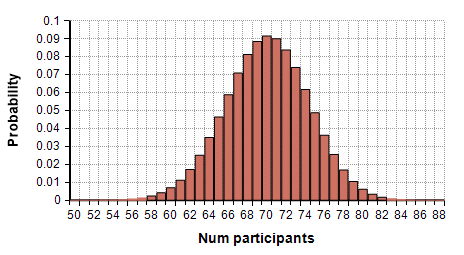# HyperGeometric distribution

(Redirected from HyperGeometric)

Release: 4.6  •  5.0  •  5.1  •  5.2  •  5.3  •  5.4  •  6.0  •  6.1  •  6.2  •  6.3  •  6.4

The hypergeometric distribution describes the number of times an event occurs in a fixed number of trials without replacement -- e.g., the number of red balls in a sample of «Trials» balls drawn without replacement from an urn containing «Size» balls of which «PosEvents» are red.

HyperGeometric( 100, 700, 1000 )## Functions

### HyperGeometric(trials, posEvents, size)

Use this to describe a variable whose outcome has a hyperGeometric distribution.

### ProbHyperGeometric(k, trials, posEvents, size)

Returns the probability of outcome «k». It is given by

$\displaystyle{ p(k) = { {\binom{posEvents}{k} \binom{size-posEvents}{trials-k} } \over \binom{size}{trials} } }$

Suppose 20 out of 30 balls in a basket are red, and you draw 10 balls at random without replacement. Then the probability of drawing exactly 7 red balls is given by:

ProbHyperGeometric(7, 10, 20, 30) → 0.3096

### CumHyperGeometric(k, trials, posEvents, size)

The cumulative probability function for the hyperGeometric distribution. Its value is equal to

$\displaystyle{ F(k) = \sum_{i=0}^{k} { {\binom{posEvents}{i} \binom{size-posEvents}{trials-i} } \over \binom{size}{trials} } }$

Use this function when computing the p-Value for a hyperGeometric statistical test.

### CumHyperGeometricInv(p, trials, posEvents, size)

The inverse cumulative probability function for the hyperGeometric distribution

### Parameters

«trials»
The sample size -— e.g., the number of balls drawn from an urn without replacement. Cannot be larger than «Size».
«posEvents»
The total number of successful events in the population -- e.g, the number of red balls in the urn.
«size»
The population size -- e.g., the total number of balls in the urn, red and non-red.